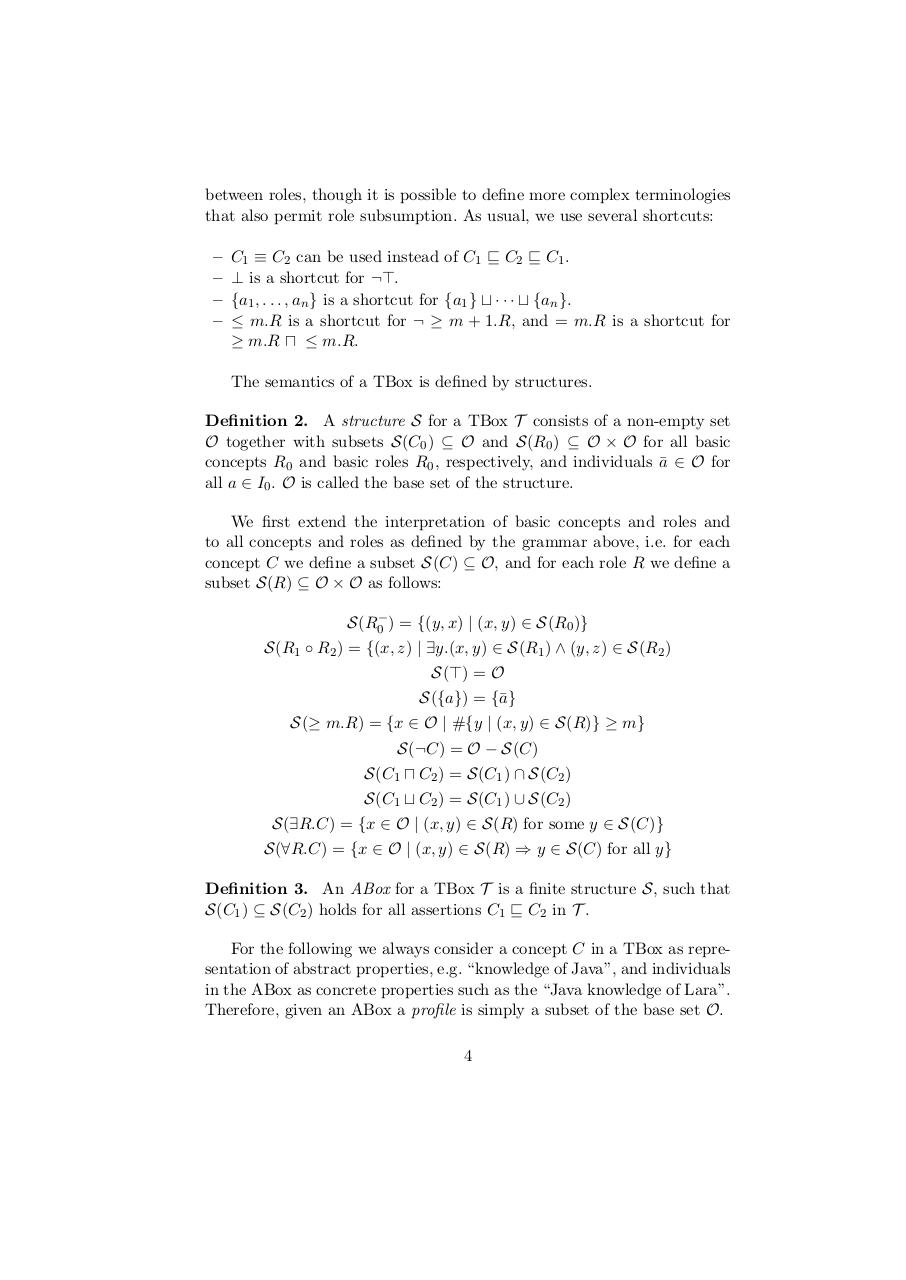# Matching Knowledge Bases.pdfPage 1 2 3 45614

#### Text preview

between roles, though it is possible to define more complex terminologies
that also permit role subsumption. As usual, we use several shortcuts:

C1 ≡ C2 can be used instead of C1 v C2 v C1 .
⊥ is a shortcut for ¬&gt;.
{a1 , . . . , an } is a shortcut for {a1 } t · · · t {an }.
≤ m.R is a shortcut for ¬ ≥ m + 1.R, and = m.R is a shortcut for
≥ m.R u ≤ m.R.
The semantics of a TBox is defined by structures.

Definition 2. A structure S for a TBox T consists of a non-empty set
O together with subsets S(C0 ) ⊆ O and S(R0 ) ⊆ O × O for all basic
concepts R0 and basic roles R0 , respectively, and individuals a
¯ ∈ O for
all a ∈ I0 . O is called the base set of the structure.
We first extend the interpretation of basic concepts and roles and
to all concepts and roles as defined by the grammar above, i.e. for each
concept C we define a subset S(C) ⊆ O, and for each role R we define a
subset S(R) ⊆ O × O as follows:
S(R0− ) = {(y, x) | (x, y) ∈ S(R0 )}
S(R1 ◦ R2 ) = {(x, z) | ∃y.(x, y) ∈ S(R1 ) ∧ (y, z) ∈ S(R2 )
S(&gt;) = O
S({a}) = {¯
a}
S(≥ m.R) = {x ∈ O | #{y | (x, y) ∈ S(R)} ≥ m}
S(¬C) = O − S(C)
S(C1 u C2 ) = S(C1 ) ∩ S(C2 )
S(C1 t C2 ) = S(C1 ) ∪ S(C2 )
S(∃R.C) = {x ∈ O | (x, y) ∈ S(R) for some y ∈ S(C)}
S(∀R.C) = {x ∈ O | (x, y) ∈ S(R) ⇒ y ∈ S(C) for all y}
Definition 3. An ABox for a TBox T is a finite structure S, such that
S(C1 ) ⊆ S(C2 ) holds for all assertions C1 v C2 in T .
For the following we always consider a concept C in a TBox as representation of abstract properties, e.g. “knowledge of Java”, and individuals
in the ABox as concrete properties such as the “Java knowledge of Lara”.
Therefore, given an ABox a profile is simply a subset of the base set O.
4